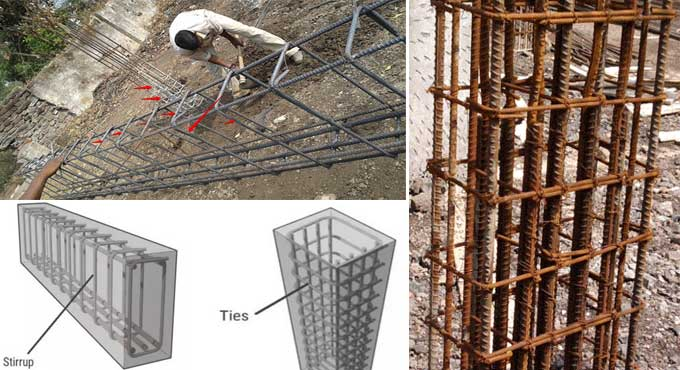# How to Make Column Stirrups in 4 Easy StepsTwo types of bars are found in an RCC column. The longitudinal bar is one, and the horizontal bar is the other. Stirrups and ties are used as horizontal bars. Construction is another area where stirrup holds some other names, reinforcements such as rings and shears. Stirrups for a column are made by calculating the length of the bar for the stirrup, determining how many stirrups to use, and determining how big the stirrup should be.

### Determine the Stirrup Size

The dimensions of the column are 20 inches by 40 inches. Column reinforcement needs a clear concrete cover of 112" in height.

As a result, 40''-2*112?=37'' and 20''-2*112?=17'' will be the length and width respectively.
Therefore, the stirrups are 37" x 17".

### Cutting Bar Length Calculations for Stirrup

Two-times the length plus two-times the width plus two-times the hook's length

This is equal to 2 x 37" + 2 x 17" + 2 x 3" = 114 inches or 9.5 feet.

Hook Length

The hook length is calculated based on a formula that is 6db multiplied by the bar diameter.

For 10mm* bar, the hook length is 6 x 10 is 60 mm.

As per code, the hook cannot be shorter than 75mm.

The stirrup hook length is 3'' (75mm = 3'').

Stirrups have a bar length of 9.5 feet. This will result in a shorter cutting bar than the actual bar. As the bar bends, its length is reduced. The reduced length of the bend is calculated using a certain formula.

The formula requires multiple calculations. 10 mm* bar - 1''

12 mm bar ? 1??

16 mm bar ? 1??

You will use a 10 mm bar to cut our stirrup, so the length of our bar will be 9.5' ? 3 x 1''. The bend deduction is not taken into account for 135? bend.= 9'-2.

### Stirrup Number Calculation

Stirrup spacing can divide into two types. S1 refers to one, and S2 refers to another. Its height is 4 inches c/c, and its width is 8 inches c/c.

The column is equipped with an S1 in the bottom 1/4th portion and the top 1/4th portion of its length. The column contains S2 in the middle of the length.

The column length is the distance between the floor and bottom of a beam.

Additionally, two stirrups for the column-to-beam joint should provide.

Let's determine how many stirrups the column should have.

10' is the height of the floor.

2' is the height of the beam.

This column has a clear height of,
Height of the beam minus the floor height
Equal to 10'-2'
That equals 8'.

### Approximately how many stirrups are there?

A stirrup should have the following dimensions: 4'/4" + 4'/8" + 2 + 1 (for accurate measurement, add just one bar).
Total is 21 nos.

### The Bar Bending in Stirrup?s Case

Machines that can make stirrups can be used for large projects requiring a great deal of them. This project requires a lot of hand-stirring.

Stirrups can be made by hand with a standard handle. Those are handcrafted stands and handles. The tools you need for making stirrups are available here.

You only need to bend the rod with the handle after putting the rod in the stand. To obtain the desired stirrup size, you must test and experiment. Make sure you also angle the hook at 135 degrees.